gru 订阅
gru，英语单词，主要用作缩写词、名词，作缩写词时译为“格勒乌，苏军总参谋部情报总局（Glavnoe Razvedivatelnoe Upravlenie）”，作名词时译为“(Gru)人名；(法)格吕”。  展开全文gru，英语单词，主要用作缩写词、名词，作缩写词时译为“格勒乌，苏军总参谋部情报总局（Glavnoe Razvedivatelnoe Upravlenie）”，作名词时译为“(Gru)人名；(法)格吕”。 

gru

GRU单词用法
ABBREVIATION for (formerly) the Soviet military intelligence service; the military counterpart of the KGB (旧)苏军总参谋部情报总局 

• ## GRU

2020-11-29 12:41:10
<p><strong>In reference , the standard GRU architecture is defined by</strong> ht=(1-zt) * ht-1+zt<em>ht </em><em>.* <p><strong>But in your paper, the standard GRU architecture is defined...
• ## gru

千次阅读 2019-01-02 16:27:57
gruGRU 中，如下图所示，只有两个门：重置门（reset gate）和更新门（update gate） RNN的一个特点是所有的隐层共享参数(U,V,W)，整个网络只用这一套参数。gru也是如此 “ * ”代表点乘 其中， rt表示重置门，...
gru
在 GRU 中，如下图所示，只有两个门：重置门（reset gate）和更新门（update gate）
RNN的一个特点是所有的隐层共享参数(U,V,W)，整个网络只用这一套参数。gru也是如此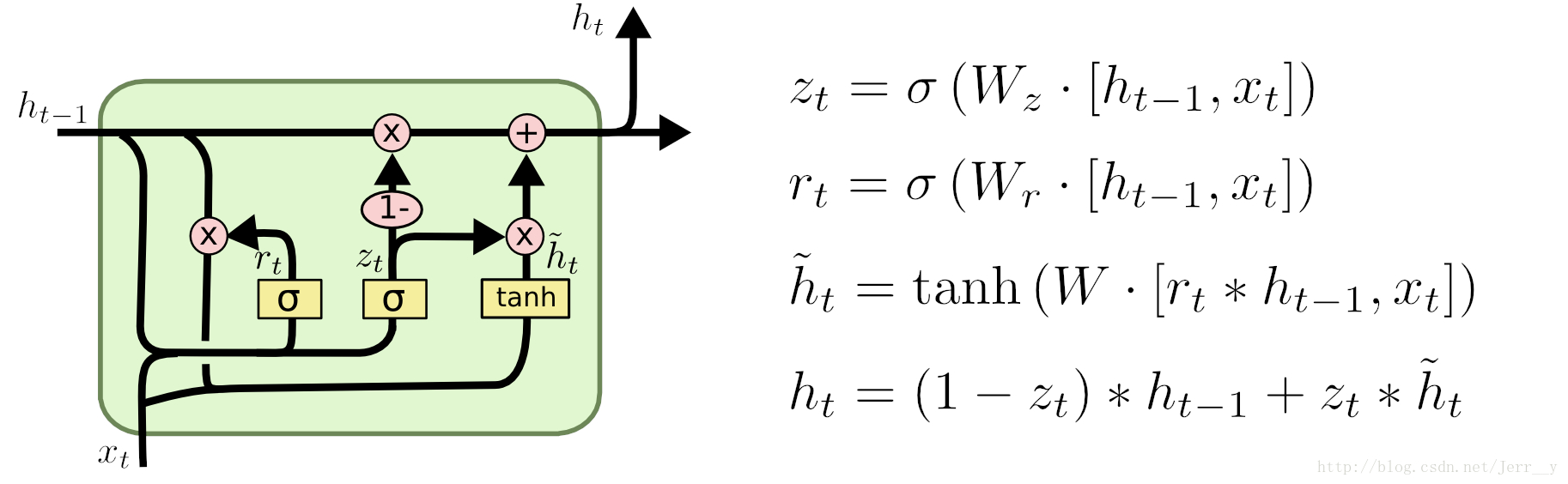“ * ”代表点乘
其中， rt表示重置门，zt表示更新门。
1重置门rt决定是否将之前的状态忘记。(作用相当于合并了 LSTM 中的遗忘门和传入门）
2将先前隐藏状态ht-1和遗忘门输出的向量进行点乘。当rt趋于0的时候，前一个时刻的状态信息ht−1会被忘掉，隐藏状态会被重置为当前输入的信息。
3得到了新的隐藏状态ĥ ， 但是还不能直接输出，而是通过更新门来控制最后的输出：ht=(1−zt)∗ht−1+zt∗ĥ t
和 LSTM 比较一下：

GRU 少一个门，同时少了细胞状态Ct。
在 LSTM 中，通过遗忘门和传入门控制信息的保留和传入；GRU 则通过重置门来控制是否要保留原来隐藏状态的信息，但是不再限制当前信息的传入。
在 LSTM 中，虽然得到了新的细胞状态 Ct，但是还不能直接输出，而是需要经过一个过滤的处理:ht=ot∗tanh(Ct)；同样，在 GRU 中, 虽然我们也得到了新的隐藏状态ĥ ， 但是还不能直接输出，而是通过更新门来控制最后的输出：ht=(1−zt)∗ht−1+zt∗ĥ t

作者：gzj_1101
来源：CSDN
原文：https://blog.csdn.net/gzj_1101/article/details/79376798
版权声明：本文为博主原创文章，转载请附上博文链接！
计算
https://www.cnblogs.com/wushaogui/p/9176617.html
假设现有
一个样本,Shape=(13,5),时间步是13,每个时间步的特征长度是5.形象点,我把一个样本画了出来:使用Keras框架添加LSTM层时,我的设置是这样的keras.layers.LSTM(10),也就是我现在设定,每个时间步经过LSTM后,得到的中间隐向量是10维(意思是5->10维),13个时间步的数据若把每部的结果输出得到的是(13，10)的数据.若只输出最后的结果就是（1，10）的数据
每个时间步对应神经元(参数)一样.计算参数时算一个时间步中参与的神经元个数即可.
先看基础 RNN 的计算公式：
h(t)=f(W[x(t),h(t−1)]+b)
Xt是新的输入，Ht-1是上一层的隐藏状态
x: [n_features, 1]([input_dimension,1])
h: [n_units, 1]（[output_dimension,1]）
[Xt,Ht-1]:[n_units+n_features, 1]
W: [n_units, n_units+n_features]
b: [n_units, 1]

rnn参数数量 = (n_features + n_units) * n_units + n_units
gru参数数量 = (n_features + n_units) * (n_units * 3) + (n_units * 3)
keras API
keras.layers.GRU(units, activation='tanh', recurrent_activation='hard_sigmoid', use_bias=True, kernel_initializer='glorot_uniform', recurrent_initializer='orthogonal', bias_initializer='zeros', kernel_regularizer=None, recurrent_regularizer=None, bias_regularizer=None, activity_regularizer=None, kernel_constraint=None, recurrent_constraint=None, bias_constraint=None, dropout=0.0, recurrent_dropout=0.0, implementation=1, return_sequences=False, return_state=False, go_backwards=False, stateful=False, unroll=False, reset_after=False)

输入尺寸
3D 张量，尺寸为 (batch_size, timesteps, input_dim) 对横向的文本，timestep就是宽。
units: 正整数，输出空间的维度。
return_sequences: 布尔值。false是返回输出序列中的最后一个输出
true是全部序列,也就是全部状态。即（batchsize，time_steps,units）o_backwards: 布尔值 (默认 False)。 如果为 True，则向后处理输入序列并返回相反的序列。（batch_size,units）


展开全文• ## GRU神经网络

万次阅读 多人点赞 2017-08-17 15:19:48
GRU是什么GRU即Gated Recurrent Unit。前面说到为了克服RNN无法很好处理远距离依赖而提出了LSTM，而GRU则是LSTM的一个变体，当然LSTM还有有很多其他的变体。GRU保持了LSTM的效果同时又使结构更加简单，所以它也非常...
前面已经详细讲了LSTM神经网络（文末有链接回去），接着往下讲讲LSTM的一个很流行的变体。

GRU是什么

GRU即Gated Recurrent Unit。前面说到为了克服RNN无法很好处理远距离依赖而提出了LSTM，而GRU则是LSTM的一个变体，当然LSTM还有有很多其他的变体。GRU保持了LSTM的效果同时又使结构更加简单，所以它也非常流行。

GRU模型

回顾一下LSTM的模型，LSTM的重复网络模块的结构很复杂，它实现了三个门计算，即遗忘门、输入门和输出门。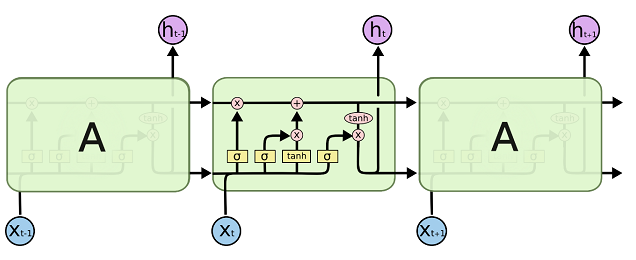而GRU模型如下，它只有两个门了，分别为更新门和重置门，即图中的zt$z_t$和rt$r_t$。更新门用于控制前一时刻的状态信息被带入到当前状态中的程度，更新门的值越大说明前一时刻的状态信息带入越多。重置门用于控制忽略前一时刻的状态信息的程度，重置门的值越小说明忽略得越多。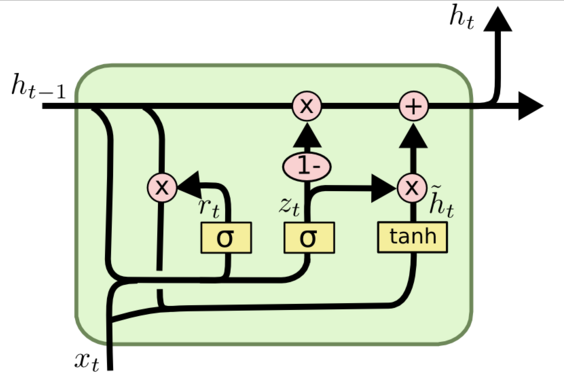GRU向前传播

根据前面GRU模型图来一步步看他是怎么向前传播的，根据图不难得到以下式子：
rt=σ(Wr⋅[ht−1,xt])r_t=\sigma(W_r\cdot[h_{t-1},x_t])

zt=σ(Wz⋅[ht−1,xt])z_t=\sigma(W_z\cdot[h_{t-1},x_t])

h~t=tanh(Wh~⋅[rt∗ht−1,xt]){\tilde{h}}_t=\tanh(W_{\tilde{h}} \cdot[r_t\ast h_{t-1},x_t])

ht=(1−zt)∗ht−1+zt∗h~th_t=(1-z_t)\ast h_{t-1}+z_t \ast \tilde{h}_t

yt=σ(Wo⋅ht)y_t = \sigma(W_o \cdot h_t)

其中[]表示两个向量相连接，*表示矩阵元素相乘。

GRU的训练

从前面的公式中可以看到需要学习的参数就是WrWzWhWo$W_r W_z W_h W_o$那些权重参数，其中前三个权重都是拼接的，所以在学习时需要分割出来，即

Wr=Wrx+WrhW_r=W_{rx}+W_{rh}

Wz=Wzx+WzhW_z=W_{zx}+W_{zh}

Wh~=Wh~x+Wh~hW_{\tilde{h}}=W_{{\tilde{h}}x}+W_{\tilde{h}h}

输出层的输入yit=Woh$y_t^i = W_oh$，输出为yot=σ(yit)$y_t^o = \sigma(y_t^i)$。

设某时刻的损失函数为Et=12(yd−yot)2$E_t = \frac{1}{2} (y_d - y_t^o)^2$，则某样本的损失为

E=∑Tt=1Et$E = \sum_{t=1}^T E_t$

与前面LSTM网络类似，最终可以推出

∂E∂Wo=δy,tht\frac{\partial{E}}{\partial{W_{o}}}=\delta_{y,t}{h}_{t}

∂E∂Wzx=δz,txt\frac{\partial{E}}{\partial{W_{zx}}}=\delta_{z,t}{x}_t

∂E∂Wzh=δz,tht−1\frac{\partial{E}}{\partial{W_{zh}}}=\delta_{z,t}{h}_{t-1}

∂E∂Wh~x=δtxt\frac{\partial{E}}{\partial{W_{\tilde{h}x}}}=\delta_{t}{x}_t

∂E∂Wh~h=δt(rt⋅ht−1)\frac{\partial{E}}{\partial{W_{\tilde{h}h}}}=\delta_{t}(r_t \cdot h_{t-1})

∂E∂Wrx=δr,txt\frac{\partial{E}}{\partial{W_{rx}}}=\delta_{r,t}{x}_t

∂E∂Wrh=δr,tht−1\frac{\partial{E}}{\partial{W_{rh}}}=\delta_{r,t}{h}_{t-1}

δy,t=(yd−yot)⋅σ′\delta_{y,t} = (y_d - y_t^o) \cdot \sigma'

δh,t=δy,tWo+δz,t+1Wzh+δt+1Wh~h⋅rt+1+δh,t+1Wrh+δh,t+1⋅(1−zt+1)\delta_{h,t} = \delta_{y,t}W_o+\delta_{z,t+1}W_{zh}+\delta_{t+1}W_{\tilde{h}h} \cdot r_{t+1} + \delta_{h,t+1}W_{rh}+ \delta_{h,t_+1} \cdot (1-z_{t+1})

δz,t=δt,h⋅(h~t−ht−1)⋅σ′\delta_{z,t} = \delta_{t,h} \cdot ({\tilde{h}}_t - h_{t-1})\cdot \sigma'

δt=δh,t⋅zt⋅ϕ′\delta_t = \delta_{h,t} \cdot z_t \cdot \phi'

δr,t=ht−1⋅[(δh,t⋅zt⋅ϕ′)Wh~h]⋅σ′\delta_{r,t} = h_{t-1} \cdot [(\delta_{h,t} \cdot z_t \cdot \phi')W_{\tilde{h}h}] \cdot \sigma'

以下是广告和相关阅读

========广告时间========

公众号的菜单已分为“分布式”、“机器学习”、“深度学习”、“NLP”、“Java深度”、“Java并发核心”、“JDK源码”、“Tomcat内核”等，可能有一款适合你的胃口。

鄙人的新书《Tomcat内核设计剖析》已经在京东销售了，有需要的朋友可以购买。感谢各位朋友。

为什么写《Tomcat内核设计剖析》

=========================

相关阅读：

LSTM神经网络

循环神经网络

卷积神经网络

机器学习之神经网络

机器学习之感知器

欢迎关注：展开全文神经网络 深度学习 人工智能 循环神经网络
• 使用双向GRU和LSTM进行FastText文本分类 # This is a script version of Meng Ye's Notebook: https://www.kaggle.com/konohayui/bi-gru-cnn-poolings/code # I'm trying to reproduce the best single public ...
• GRUGRUCell 最直观的图解 一、API 1.1 GRU 官方计算方式如下： rt=σ(Wirxt+bir+Whrh(t−1)+bhr)zt=σ(Wizxt+biz+Whzh(t−1)+bhz)nt=tanh⁡(Winxt+bin+rt∗(Whnh(t−1)+bhn))ht=(1−zt)∗nt+zt∗h(t−1) r_t = \...
GRU和GRUCell
最直观的图解一、API
1.1 GRU
官方计算方式如下：
$r_t = \sigma(W_{ir} x_t + b_{ir} + W_{hr} h_{(t-1)} + b_{hr}) \\ z_t = \sigma(W_{iz} x_t + b_{iz} + W_{hz} h_{(t-1)} + b_{hz}) \\ n_t = \tanh(W_{in} x_t + b_{in} + r_t * (W_{hn} h_{(t-1)}+ b_{hn})) \\ h_t = (1 - z_t) * n_t + z_t * h_{(t-1)}$
API如下
nn.GRU(*args, **kwargs):

input_size: 输入的每一维的特征数（或许叫做feature_num比较容易理解）
hidden_size: The number of features in the hidden state h
num_layers: Number of recurrent layers. E.g., setting num_layers=2
would mean stacking two GRUs together to form a stacked GRU,
with the second GRU taking in outputs of the first GRU and
computing the final results. Default: 1
bias: If False, then the layer does not use bias weights b_ih and b_hh.
Default: True
batch_first: If True, then the input and output tensors are provided
as (batch, seq, feature). Default: False
dropout: If non-zero, introduces a Dropout layer on the outputs of each
GRU layer except the last layer, with dropout probability equal to
:attr:dropout. Default: 0
bidirectional: If True, becomes a bidirectional GRU. Default: False

示例如下：
import torch
import torch.nn as nn

rnn = nn.GRU(input_size=10, hidden_size=20, num_layers=2,batch_first=True)
# (batch_size, seq_len, input_size)
input = torch.randn(3 , 5 , 10)
# (num_layers * num_directions, batch, hidden_size)
h0 = torch.randn(2, 3, 20)

# output shape :[5, 3, 20]
output, hn = rnn(input, h0)

1.2 GRUCell
计算表达式：
$r = \sigma(W_{ir} x + b_{ir} + W_{hr} h + b_{hr}) \\ z = \sigma(W_{iz} x + b_{iz} + W_{hz} h + b_{hz}) \\ n = \tanh(W_{in} x + b_{in} + r * (W_{hn} h + b_{hn})) \\ h' = (1 - z) * n + z * h$
是不是很眼熟，是不是感觉和上面的形式是一模一样的，他们本来就是一个东西，只是一个GRU可以有多个GRUCell,经过多个时间步组合起来的GRUCell就可以看成是GRU了。
函数的签名（意义和GRU一致，不重复了，手动狗头）：
nn.GRUCell(input_size, hidden_size, bias=True)

实例如下：
import torch
import torch.nn as nn

rnn = nn.GRUCell(10, 20)

# (_,batch_size.input_size)
input = torch.randn(6, 3, 10)

# (batch, hidden_size)
hx = torch.randn(3, 20)
output = []
for i in range(6):
hx = rnn(input[i], hx)
output.append(hx)
# 6
print(len(output))

# (batch, hidden_size)=[3, 20]
output.shape

二、使用以及区别
其实在上面已经表明，经过多个时间步组合起来的GRUCell就可以看成是GRU了。但是为什么有了GRU还会有GRUCell呢，明明已经封装的这么好了，干嘛要再拆开？
可能是另外有大用处吧，个人用的GRU较多，基本没怎么用GRUCell,如果后续有到确定要用GRUCell的情况会再来补充。
Reference
nn.GRU
nn.GRUCell


展开全文神经网络
• GRU-ARIMA时间序列预测 GRU和ARIMA模型用于时间序列预测，其中GRU可用于短期和长期预测。使用GRU和ARIMA模型进行时间序列预测，其中GRU可以进行短期预测和长期预测。
• <div><ol><li>Support <code>linear_before_reset</code> by implementing a different GRU with that option</li><li>Fix errors using bidirectional GRU</li></ol>该提问来源于开源项目：onnx/onnx-...
• pytorch GRU 一、GRU简介 图中的和分别表示更新门和重置门。更新门用于控制前一时刻的状态信息被带入到当前状态中的程度，更新门的值越大说明前一时刻的状态信息带入越多。重置门控制前一状态有多少信息被写入到...


pytorch GRU

目录

pytorch GRU

一、GRU简介1

二、GRU简介2

三、pytorch GRU

3.1    定义GRU ()

3.2    定义好GRU()后参数，这里不明白，可先看下面实例，再回头看此项：

3.3    计算公式

3.4    实例：

一、GRU简介1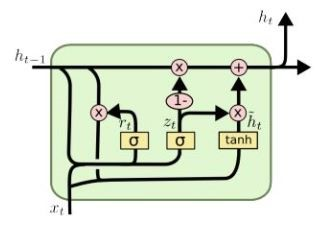图中的和分别表示更新门和重置门。更新门用于控制前一时刻的状态信息被带入到当前状态中的程度，更新门的值越大说明前一时刻的状态信息带入越多。重置门控制前一状态有多少信息被写入到当前的候选集上，重置门越小，前一状态的信息被写入的越少。

具体计算公式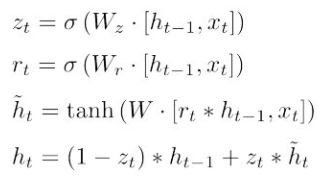二、GRU简介2

可以将GRU看成一个基本的神经单元；每个GRU单元输入为、，输出为、；具体见下图：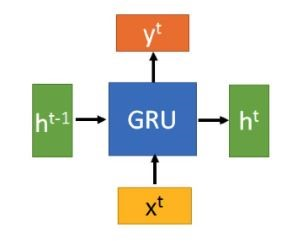三、pytorch GRU

3.1    定义GRU ()

torch.nn. GRU ()

参数为：
input_size: 输入x的特征维度，实际为词向量的维度。

hidden_size:隐藏状态 h特征的个数，实际为隐藏神经元的个数。

num_layers: GRU的层数，默认值为1。

bias: 默认值为 True ；取 False ，既不使用偏置项。

batch_first: 默认值为 False 。表示input和output张量的形式，默认是 False，就是这样形式，(seq, batch, feature)，也就是将序列长度放在第一位，batch 放在第二位；如果是True，input和output张量的形式为 (batch, seq, feature)

dropout: 默认值为0 。

bidirectional: 默认值为False，即为单向GRU； 取 True ，即为双向GRU。

3.2    定义好GRU()后参数，这里不明白，可先看下面实例，再回头看此项：

输入项
Inputs: input, h_0

input: input各维度内容所代表的的含义 (seq_len, batch, input_size)，seq_len表示句子的长度，batch每个batch包含句子的个数，input_size每个词向量的维度。

h_0: h_0不一定非要输入。h_0各维度内容所代表的的含义(num_layers * num_directions, batch, hidden_size)，num_layers表示隐藏层神经元的个数，num_directions 表示RNN的方向数，batch每个batch包含句子的个数，hidden_size隐藏层神经元的个数。

输出项

Outputs: output, h_n

output: 各维度内容所代表的的含义(seq_len, batch, num_directions * hidden_size)。

h_n: 各维度内容所代表的的含义(num_layers * num_directions, batch, hidden_size)。

3.3    计算公式

计算公式为：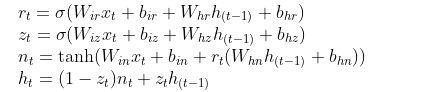第K层GRU单元的参数维度为

weight_ih_l[k]:第K层 (W_ir|W_iz|W_in) 的维度为(3*hidden_size x input_size)

bias_ih_l[k]:第K层(b_ir|b_iz|b_in)维度为(3*hidden_size)。

weight_hh_l[k]: 第K层(W_hr|W_hz|W_hn)维度为(3*hidden_size x hidden_size)

bias_hh_l[k]: 第K层(b_hr|b_hz|b_hn)维度为(3*hidden_size)

使用如下公式对所有参数进行初始化：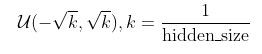3.4    实例：

import torch
import torch.nn

# 构造RNN网络，x的维度5，隐层的维度10,网络的层数2
gru_ = torch.nn.GRU(input_size=5, hidden_size=10,
num_layers =3, bias=True,
batch_first=False,
dropout=0, bidirectional=True)

# inputs:(seq_len, batch, input_size),
#  构造一个输入序列，长为 6，batch 是 3， 特征是 5
inputs = Variable(torch.randn(6, 3, 5))

#h_0:(num_layers*num_directions, batch, hidden_size)
#c_0:(num_layers*num_directions, batch, hidden_size)
h_0 = torch.randn(3*2, 3, 10)

# h0可以指定或者不指定
out, h_t = gru_(inputs, h_0)

#output:(seq_len, batch, num_directions * hidden_size)
print('out.shape: ', out.shape)
#h_t:(num_layers * num_directions, batch, hidden_size)
print('h_t.shape: ', h_t.shape)


运行结果：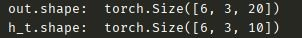`
展开全文• 循环神经网络GRU模型Matlab 代码，可直接运行，用于学习。
• GRU（Gated Recurrent Unit）是Cho在2014年提出，为LSTM的一个变种，GRU保持了LSTM的效果、结构更加简单易于训练，同时也可以解决长短时记忆和梯度问题。 在LSTM中引入了三个门函数：输入门、遗忘门和输出门来控制...深度学习
• 带有Attn-GRU的NMT 专注GRU的神经机器翻译Python
• ## GRU网络

千次阅读 2019-04-03 15:44:21
GRU网络 简介 随着 LSTM 在自然语言处理特别是文本分类任务的广泛应 用，人们逐渐发现 LSTM 具有训练时间长、参数较多、内部计 算复杂的缺点。Cho 等人在 2014 年进一步提出了更加简单的、 将 LSTM 的单元状态和隐...LSTM
• import torch import torch.nn as nn class GRU(nn.Module): '''GRU + 全连接''' def __init__(self, num_layers=1, input_size=8, hidden_size=64, time_step=20, output_size=1): ...Pytorch...